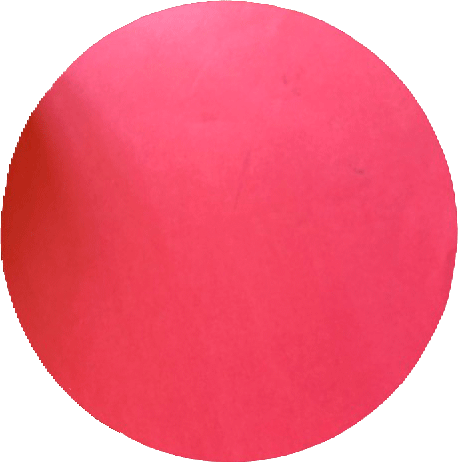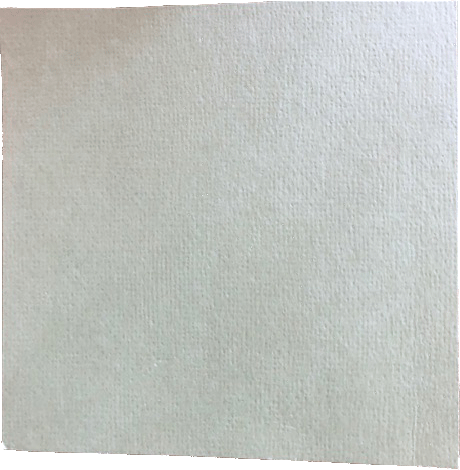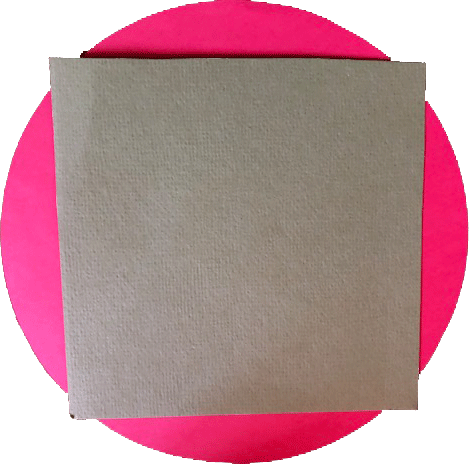SEARCH HOMEMath Central Quandaries & QueriesQuestion from a student: given a circle and square of same perimeter, why does the circle have a larger area I'm looking for an intuitive explanation rather than a computational one. ThanksHi,

I can give you a construction argument. I'm not sure how intuitive it is but it has no arithmetic calculations.

First I traced the circular base of a 3.36 L paint can and cut it out.Next I wrapped a length of string around the can so that the string length was the length of the perimeter of the circle. I folded the string in half and then in half again to obtain the length of a side of a square with the same perimeter as the circle. I constructed a square with this side length and cut it out.Finally I placed the square on top of the circle. Which has the larger area?Try it. You need a reasonably large circle to see the difference,
HarleyMath Central is supported by the University of Regina and The Pacific Institute for the Mathematical Sciences.